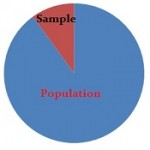# Non-Probability Sampling: Definition, Types

Share on

## What is Non-Probability Sampling?Non-probability sampling is a sampling technique where the probability of any member being selected for a sample cannot be calculated. It’s the opposite of probability sampling, where you can calculate the probability. In addition, probability sampling involves random selection, while non-probability sampling does not—it relies on the subjective judgement of the researcher.

The probabilities do not have to be equal for a method to be considered probability sampling. For example, one person could have a 10% chance of being selected and another person could have a 50% chance of being selected. It’s non-probability sampling when you can’t calculate the probabilities at all.

Watch the video for an overview of the different types of non-probability sampling:

A major advantage with non-probability sampling is that—compared to probability sampling—it’s very cost- and time-effective. It’s also easy to use and can also be used when it’s impossible to conduct probability sampling (e.g. when you have a very small population to work with).

One major disadvantage of non-probability sampling is that it’s impossible to know how well you are representing the population. Plus, you can’t calculate confidence intervals and margins of error. This is the major reason why, if at all possible, you should consider probability sampling methods first.

Despite the disadvantages, survey data collection costs have risen dramatically in recent years, resulting in many researchers and polling companies abandoning expensive probability-based samples for less expensive non-probability methods (Wisniowski et al., 2020).

## Types of Non-Probability Sampling

• Convenience Sampling: as the name suggests, this involves collecting a sample from somewhere convenient to you: the mall, your local school, your church. Sometimes called accidental sampling, opportunity sampling or grab sampling.
• Haphazard Sampling: where a researcher chooses items haphazardly, trying to simulate randomness. However, the result may not be random at all and is often tainted by selection bias.
• Purposive Sampling: where the researcher chooses a sample based on their knowledge about the population and the study itself. The study participants are chosen based on the study’s purpose. There are several types of purposive sampling. For a full list, advantages and disadvantages of the method, see the article: Purposive Sampling.
• Expert Sampling: in this method, the researcher draws the sample from a list of experts in the field.
• Heterogeneity Sampling / Diversity Sampling: a type of sampling where you deliberately choose members so that all views are represented. However, those views may or may not be represented proportionally.
• Modal Instance Sampling: The most “typical” members are chosen from a set.
• Quota Sampling: where the groups (i.e. men and women) in the sample are proportional to the groups in the population.
• Snowball Sampling: where research participants recruit other members for the study. This method is particularly useful when participants might be hard to find. For example, a study on working prostitutes or current heroin users.

## References

Dodge, Y. (2008). The Concise Encyclopedia of Statistics. Springer.
Everitt, B. S.; Skrondal, A. (2010), The Cambridge Dictionary of Statistics, Cambridge University Press.
Wisniowski, A. et al. Integrating Probability and Nonprobability Samples for Survey Inference. Journal of Survey Statistics and Methodology, Volume 8, Issue 1, February 2020, Pages 120–147, https://doi.org/10.1093/jssam/smz051

CITE THIS AS:
Stephanie Glen. "Non-Probability Sampling: Definition, Types" From StatisticsHowTo.com: Elementary Statistics for the rest of us! https://www.statisticshowto.com/probability-and-statistics/sampling-in-statistics/non-probability-sampling/
---------------------------------------------------------------------------Need help with a homework or test question? With Chegg Study, you can get step-by-step solutions to your questions from an expert in the field. Your first 30 minutes with a Chegg tutor is free!